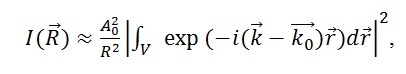# Abstract P-49: Determination of Relative Positions of Two Helices in Two-Helical Proteins Basing on X-ray Diffraction Data with the Use of Convolutional Neural Networks

Valery Novoseletsky, Mikhail Lozhnikov, Grigoriy Armeev, Alexandr Kudriavtsev, Alexey Shaytan, Georgy Kobelkov, Konstantin Shaitan

International Journal of Biomedicine.2019;9  Suppl_1:S39-S39.
http://dx.doi.org/10.21103/IJBM.9.Suppl_1.P49
Originally published June 29, 2019

Abstract:

Background: Protein structure determination using X-ray free-electron laser (XFEL) includes analysis and merging a large number of snapshot diffraction patterns.  Convolutional neural networks are widely used to solve numerous computer vision problems, e.g. image classification, and can be used for diffraction pattern analysis. But the task of protein structure determination with the use of CNNs only is not yet solved.
Methods: We collected a number of predominantly alpha-helical protein structures from PDB and analyzed their geometry. Relatively straight helices were left unchanged while curved ones were split into helices of smaller length. Finally, 88 two-helical protein structures were selected with the length of helices from 5 to 38 residues (7 to 57Å). For every structure radii, lengths and relative position and orientation of helices were calculated.
Diffraction patterns were calculated by means of straight modeling. Every structure was approximated as a pair of cylinders of given length and radius and then its diffraction image was calculated with the explicit formula:where I(R) is intensity generated on the point of detector with radius-vector R, V is the volume of structure, A0 is an amplitude of w-ray wave, k0 is a vector of initial wave, k is a vector of scattered wave. The obtained collection of diffraction patterns was used to train and test the convolutional neural network (CNN). A number of convolutional layers is used to extract features from input images. Then, a dense layer is used to solve a multi-class classification problem. In order to obtain learnable parameters, we have to solve the minimization problem of the cross-entropy loss function.
Results: Preliminary length and radius of helices with given sequence could be obtained from molecular modeling. Taking this into account, our model demonstrates a possibility to classify helix pairs into up to 50 disjoint classes.
Conclusion: CNNs could be successively used for the purpose of classification of two-helical idealized protein structures. This could be used for preliminary analysis of protein conformation. Our further efforts will be directed towards enlarging the number of classes and expanding our approach to more complex structures.